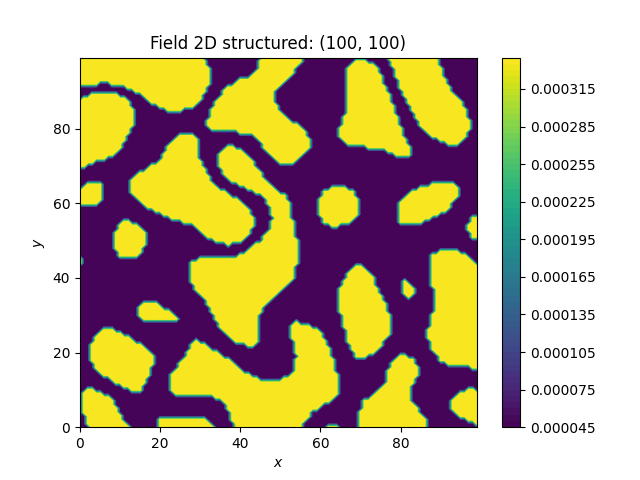# Combinations¶

You can combine different transformations simply by successively applying them.

Here, we first force the single field realization to hold the given moments, namely mean and variance. Then we apply the Zinn & Harvey transformation to connect the low values. Afterwards the field is transformed to a binary field and last but not least, we transform it to log-values.

```import gstools as gs

# structured field with a size of 100x100 and a grid-size of 1x1
x = y = range(100)
model = gs.Gaussian(dim=2, var=1, len_scale=10)
srf = gs.SRF(model, mean=-9, seed=20170519)
srf.structured([x, y])
gs.transform.normal_force_moments(srf)
gs.transform.zinnharvey(srf, conn="low")
gs.transform.binary(srf)
gs.transform.normal_to_lognormal(srf)
srf.plot()
```The resulting field could be interpreted as a transmissivity field, where the values of low permeability are the ones being the most connected and only two kinds of soil exist.

Total running time of the script: ( 0 minutes 1.108 seconds)

Gallery generated by Sphinx-Gallery